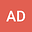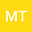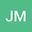Improving probability distribution estimation accuracy using polynomial approaches with the method of moments
•••Meltem Turan
Ege Universitesi Matematik Bolumu
Author ProfileJoakim Munkhammar
Uppsala universitet Institutionen for teknikvetenskaper
Author Profile## Abstract

Moment-based determination of a probability density function (PDF) is widely used in chemical and process engineering applications as they provide an efficient approach to analyze and solve complex systems. In this study, three different approaches are implemented for estimating probability distributions from their moments. These procedures are based on the reconstruction of a distribution knowing only a limited number of moments using Chebyshev polynomials, Hermite polynomials and Lagrange polynomials. To show the applicability of these approaches, various test cases with reduced set of moments are solved with a set of standard distribution families (Normal, Weibull, Log Normal and Bimodal) and with complex distributions (Smoluchowski coagulation equation and population balance equation). The results are compared with their analytical solutions using both small and big variance distributions. The Kolmogorov-Smirnov (K-S) matrix and the RMSE values of the interpolation procedure using Lagrange polynomials predict a better estimation for all the test cases compared to the other approaches including the standard monomial approach (with higher number of moments) implemented in this study. The K-S test values decreased by 19% compared to the standard monomial procedure and 11% compared to both Chebyshev and Hermite polynomial approaches. Similarly, the RMSE values improved by 85% compared to the standard monomial procedure and 62% compared to both Chebyshev and Hermite polynomial approaches. This indicates that the procedure using Lagrange polynomials is a more reliable reconstruction procedure that calculates the approximate distribution using lesser number of moments N which is desirable.